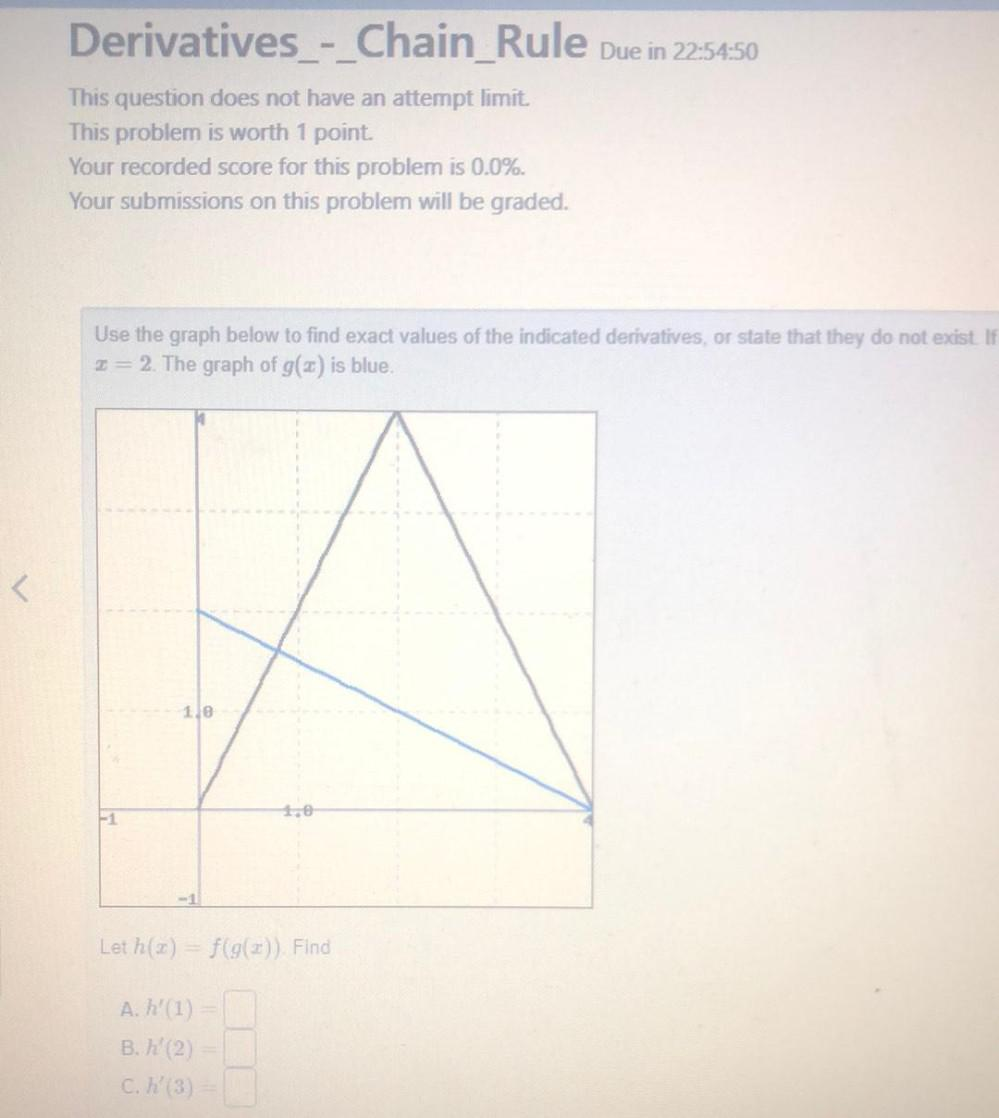Question:

# Derivatives - Chain_Rule Due in 22:54:50 This question does not have an attempt limit. This problem is worth 1 point. Your recorDerivatives - Chain_Rule Due in 22:54:50 This question does not have an attempt limit. This problem is worth 1 point. Your recorded score for this problem is 0.0%. Your submissions on this problem will be graded. Use the graph below to find exact values of the indicated derivatives, or state that they do not exist. If I= 2. The graph of g() is blue. < 1.0 1.0 Let h(2) = f(g(2)) Find A. h' (1) B. h' (2) C. h (3